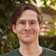# Leetcode - Episode 10 - Short Solutions (1 x M, 2 x E)

January 10, 2019

I’m really learning a lot by reading the discussion boards after submitting each of my near-optimal solutions — especially, optimization. I’m also noticing holes in my toolset. E.g., bitwise — as we’ll see in just a second.

This Leetcode series started after I felt like I wasn’t getting that much practical knowledge going over algorithm lectures and books. I knew enough of the basics to start putting solving and basic analysis into practise .. so I did. Solving three problems everyday increase my producivity as well which is a huge bonus.

The most beneficial path to me at this point is to use both types of resources.

Since I have a fixed number that I aim for each day, I am finding myself shying away to the more simple problems but this isn’t nesscarily a bad thing. The problems, the research, and the post-solve reading serve as building blocks for taking on the harder ones.

### 137. Single Number II

Problem: Given an array of integers, find the only element that doesn’t appear three times.

I knew that the most optimal solution to this problem was going to be by bitwise methods but it wasn’t coming to me. My bitwise capabilities feel below par.

I attempted to solve it as best as I could and ended up with a neat linear-time program.

``````from collections import Counter
class Solution:
def singleNumber(self, nums):
"""
:type nums: List[int]
:rtype: int
"""
ints = Counter()
maybes = set()
for i in nums:
ints[i] += 1
if ints[i] == 3:
maybes.remove(i)
for i in maybes:
return i``````

For every element in the array, there are a few more Dictionary operations than one might like but they are all constant time, and the code is clean and precise.

Runtime complexity: `O(n)`.

Spacetime complexity: `O(n)`.

### 509. Fibonacci Number

Problem: Given `n` find the `n`th Fibonacci number.

It’s a shame that this problem doesn’t support memoization because that would make it a little more interesting.

As it is, the only mistep you can make is going for a heavy-handed recursive solution. Mine just iterates and adds.

``````class Solution:
def fib(self, N):
"""
:type N: int
:rtype: int
"""
if N == 0:
return 0

last = 1
curr = 1
i = 2
while i < N:
temp = curr
curr += last
last = temp
i += 1
return curr``````

A fairly neat solution. Only beaten if you use math which brings a constant runtime. E.g., using a formula for the n^th Fibonacci sequence in terms of the golden ratio.

Runtime complexity: `O(n)`.

Space complexity: `O(1)`.

### 383. Ransom Note

Problem: Given a ransom note string and a magazine string work out whether the ransom note can be constructed from the magazine.

I first attempted to solve this with a pair of Dictionaries, then a single Dictionary, then I remembered that when dealing with single letters it’s usual viable to use an array instead.

``````import string
class Solution(object):
def canConstruct(self, ransomNote, magazine):
"""
:type ransomNote: str
:type magazine: str
:rtype: bool
"""
letters =  * 26
for i in magazine:
letters[string.lowercase.index(i)] += 1

for i in ransomNote:
letters[string.lowercase.index(i)] -= 1
if letters[string.lowercase.index(i)] < 0:
return False

return True``````

Mostly pretty simple solutions today. I worked through some tree problems but ran into some edge cases. Presumably at some point I will post up a whole swathe of tree and graph problems!

Runtime complexity: `O(n)`.

Spacetime complexity: `O(1)`.